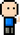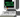# Josh Meets Computer# Support Vector Machine

April 30, 2018

Support Vector Machine is a machine learning model that makes use of a hinge loss function (and usually a regularizer component) to optimize a linear classification model. It’s best described by it’s loss function, which seeks to create a margin between the score it gives to the correct label, and the score it gives to the other incorrect labels.

$$\large Li = \sum{j \neq y} max(0, sj - s{y_i} + \Delta)$$

The loss function can be described as the sum of differences between the prediction score given to the wrong class ($sj$) and the prediction score of the correct class($s{y_i}$), only if the prediction score of the wrong class was higher by a defined margin($\Delta$). The cost function wants all the scores assigned to the wrong class to be lower than the correct class by $\Delta$. The fact that we only care about differences greater than zero (max function) makes this a hinge loss function.

Alternatives are squared hinge loss ($\max(0, -)^2$) which would penalize a boundary that allows data points to cross the margin more strongly.

## Regularization

If there is a transformation $f(xi) = Wixi + b$ where the prediction $f(xi)$ scores correctly (therefore generating zero loss), then any scaling of $W$ would yield the same loss of zero. This can cause the weights to explode to large numbers, so we want to penalize overly large weights.

This technique also prevents overfitting, where the model may begin to favor a specific feature and begin maxing out a specific weight over all others. Adding this L2 regularization improves generalization of the model.

$$\large R(W) = \lambda \sum{k}\sum{l}W^2_{k,l}$$

import numpy as np

class SVM():

def __init__(self, num_features, num_classes, e=1):
self.features = num_features
self.labels = num_classes
self.params = {}

self.params['W'] = np.random.rand(self.features, self.labels) * e  # so it's not zero initialization
self.params['b'] = np.zeros((self.labels))
self.params['dW'] = np.zeros((self.features, self.labels))
self.params['loss'] = 0

def evaluate(self, X, y, delta=1, reg=0.005):
num_examples = X.shape

assert X.shape == self.features, "input shape does not match feature size of model"

# Forward pass
W = self.params.get('W')
b = self.params.get('b', np.zeros((num_examples, 1)))
hypothesis = np.dot(X, W) + b

# One hot encode y class labels
one_hot = np.eye(self.labels)[y.reshape(-1)]
correct_scores = hypothesis * one_hot

# Hinge Loss
diff = hypothesis - correct_scores + delta
diff -= diff * one_hot
cost = np.maximum(np.zeros_like(diff), diff)  # features x labels

# Regularization
L2 = 0.5 * reg * np.sum(W**2)

# SVM
self.params['loss'] = 1 / num_examples * np.sum(cost) + L2

# backward pass
grad[diff > 0] = 1  # wrong scores to 1
diff_total = np.sum(grad, axis=1) # sum wrong scores for each example

self.params['dW'] = 1 / num_examples * np.dot(X.T, grad) + reg * W

def fit(self, X, y, epochs=50, batch=20, lr = 1e-2, lr_decay=0.9):
num_train=X.shape
iters_per_epoch = max(num_train // batch, 1)

loss_hist = []

for e in range(epochs):
for it in range(iters_per_epoch):
# Select random [batch size] samples from data
batch_idx = np.random.choice(X.shape, 10, replace=False)

X_batch = X[batch_idx]
Y_batch = y[batch_idx]

# Evaluate -- new loss and grad
self.evaluate(X_batch, Y_batch)

# Update weights
self.params['W'] -= lr * self.params['dW']
self.params['b'] -= lr * self.params['db']
loss_hist.append(self.params['loss'])

if it % iters_per_epoch == 0:
lr *= lr_decay  # Learning Rate Decay
return loss_hist

def predict(self, X):
W = self.params.get('W')
b = self.params.get('b', np.zeros((num_examples, 1)))
return np.dot(X, W) + b

%matplotlib inline
import numpy as np
import matplotlib.pyplot as plt
from sklearn import svm, datasets

# import some data to play with
X = iris.data[:, :2]  # we only take the first two features.
y = iris.target
h = .02  # step size in the mesh

# create a mesh to plot in
x_min, x_max = X[:, 0].min() - 1, X[:, 0].max() + 1
y_min, y_max = X[:, 1].min() - 1, X[:, 1].max() + 1
xx, yy = np.meshgrid(np.arange(x_min, x_max, h),
np.arange(y_min, y_max, h))

# Plot also the training points
fig =plt.figure(figsize=(10,6))
plt.scatter(X[:, 0], X[:, 1], c=y, cmap=plt.cm.coolwarm, linewidth=1, edgecolor='black')
plt.xlabel('Sepal length')
plt.ylabel('Sepal width')
plt.xlim(xx.min(), xx.max())
plt.ylim(yy.min(), yy.max())
plt.xticks(())
plt.yticks(())
plt.title('Data')
plt.show()
# Scale Data
x_scaled = X.copy()
mean = X.min(axis=0)
stdd = X.std(axis=0)
x_scaled -= mean
x_scaled /= stdd

# Create and Train Model
svm = SVM(2, 3)
loss = svm.fit(x_scaled, y, lr_decay=0.99)

# Plot Loss
fig = plt.figure(figsize=(10,6))
plt.show()